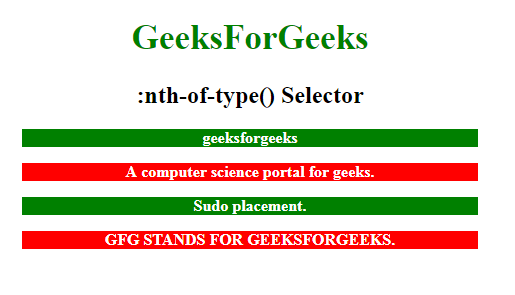# CSS | :nth-of-type() Selector

• Last Updated : 12 Apr, 2019

The :nth-of-type() in css Selector is used to style only those elements which are the nth number of child of its parent element. An n may be a number, a keyword, or a formula.

Syntax:

```:nth-of-type(number) {
// CSS Property;
} ```

Where number is the argument that represents the pattern for matching elements . It can be odd, even or in a functional notation.

• odd: It represents elements whose position is odd in a series: 1, 3, 5, etc., counting from the end.
• even: It represents the elements whose position is even in a series: 2, 4, 6, etc., counting from the end.
• functional notation (): It represents elements whose position of siblings matches the pattern An+B, for every positive integer or zero value of n. The index of the first element, counting from the end, is 1.

Example-1:

 ```<``html``>`` ` `<``head``>``    ``<``style``>``        ``h1 {``            ``color: green;``            ``font-size: 35px;``        ``}``         ` `        ``p:nth-of-type(2) {``            ``background: green;``            ``color: white;``            ``font-weight: bold;``            ``width: 70%;``        ``}``    `````` ` `<``body``>``    ``<``center``>``        ``<``h1``>GeeksForGeeks``        ``<``h2``>:nth-of-type() Selector ``        ``<``p` `class``=``"geeksFORGEEKS"``>geeksforgeeks``            ``<``p` `class``=``"forgeeks"``>A computer science portal for geeks.``                ``<``p` `class``=``"geeks"``>Sudo placement.``                ``<``p` `class``=``"SUDO"``>GFG STANDS FOR GEEKSFORGEEKS.`` ` ``` ` ``

Output :Example-2: In this Example every odd and even element is selected by using the odd or even keyword as an argument.

 ```<``html``>`` ` `<``head``>``    ``<``style``>``        ``h1 {``            ``color: green;``            ``font-size: 35px;``        ``}``         ` `        ``p:nth-of-type(odd) {``            ``background: green;``            ``color: white;``            ``font-weight: bold;``            ``width: 70%;``        ``}``         ` `        ``p:nth-of-type(even) {``            ``background: red;``            ``color: white;``            ``font-weight: bold;``            ``width: 70%;``        ``}``    `````` ` `<``body``>``    ``<``center``>``        ``<``h1``>GeeksForGeeks``        ``<``h2``>:nth-of-type() Selector ``        ``<``p` `class``=``"geeksFORGEEKS"``>geeksforgeeks``            ``<``p` `class``=``"forgeeks"``>A computer science portal for geeks.``                ``<``p` `class``=``"geeks"``>Sudo placement.``                ``<``p` `class``=``"SUDO"``>GFG STANDS FOR GEEKSFORGEEKS.`` ` ``` ` ``

output:Supported Browsers: The browser supported by :nth-of-type() Selector are listed below:

• Apple Safari